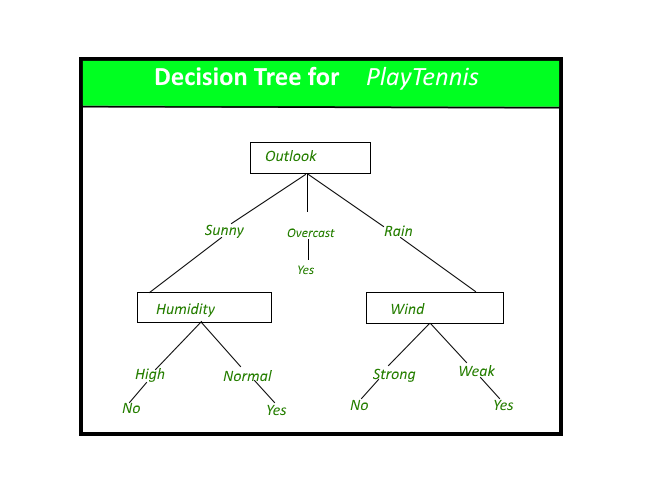# Decision Tree

Decision Tree : Decision tree is the most powerful and popular tool for classification and prediction. A Decision tree is a flowchart like tree structure, where each internal node denotes a test on an attribute, each branch represents an outcome of the test, and each leaf node (terminal node) holds a class label.A decision tree for the concept PlayTennis.

Construction of Decision Tree :
A tree can be “learned” by splitting the source set into subsets based on an attribute value test. This process is repeated on each derived subset in a recursive manner called recursive partitioning. The recursion is completed when the subset at a node all has the same value of the target variable, or when splitting no longer adds value to the predictions. The construction of decision tree classifier does not require any domain knowledge or parameter setting, and therefore is appropriate for exploratory knowledge discovery. Decision trees can handle high dimensional data. In general decision tree classifier has good accuracy. Decision tree induction is a typical inductive approach to learn knowledge on classification.

Decision Tree Representation :
Decision trees classify instances by sorting them down the tree from the root to some leaf node, which provides the classification of the instance. An instance is classified by starting at the root node of the tree,testing the attribute specified by this node,then moving down the tree branch corresponding to the value of the attribute as shown in the above figure.This process is then repeated for the subtree rooted at the new node.

The decision tree in above figure classifies a particular morning according to whether it is suitable for playing tennis and returning the classification associated with the particular leaf.(in this case Yes or No).
For example,the instance

(Outlook = Rain, Temperature = Hot, Humidity = High, Wind = Strong )

would be sorted down the leftmost branch of this decision tree and would therefore be classified as a negative instance.

In other words we can say that decision tree represent a disjunction of conjunctions of constraints on the attribute values of instances.

(Outlook = Sunny ^ Humidity = Normal) v (Outllok = Overcast) v (Outlook = Rain ^ Wind = Weak)

Strengths and Weakness of Decision Tree approach
The strengths of decision tree methods are:

• Decision trees are able to generate understandable rules.
• Decision trees perform classification without requiring much computation.
• Decision trees are able to handle both continuous and categorical variables.
• Decision trees provide a clear indication of which fields are most important for prediction or classification.

The weaknesses of decision tree methods :

• Decision trees are less appropriate for estimation tasks where the goal is to predict the value of a continuous attribute.
• Decision trees are prone to errors in classification problems with many class and relatively small number of training examples.
• Decision tree can be computationally expensive to train. The process of growing a decision tree is computationally expensive. At each node, each candidate splitting field must be sorted before its best split can be found. In some algorithms, combinations of fields are used and a search must be made for optimal combining weights. Pruning algorithms can also be expensive since many candidate sub-trees must be formed and compared.

References :
Machine Learning, Tom Mitchell, McGraw Hill, 1997.

In the next post we will be discussing about ID3 algorithm for the construction of Decision tree given by J. R. Quinlan.

This article is contributed by Saloni Gupta. If you like GeeksforGeeks and would like to contribute, you can also write an article using contribute.geeksforgeeks.org or mail your article to contribute@geeksforgeeks.org. See your article appearing on the GeeksforGeeks main page and help other Geeks.